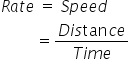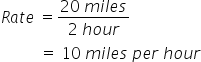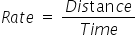Mathematics
Easy

Question

# Pete roller skates 20 miles in 2 hours. At this rate, time taken by him to cover 35 miles is ___________ hours.

## 2.53.13.52.7Hint:

## The correct answer is: 3.5

### The time required by Pete to roller skate the distance of 20 miles is 2 hours.Using this data, we can find the rate at which Pete is roller skating.Rate means the speed at which Pete is roller skating. Once we find the rate, we can use it to find the time required to cover any distance, given the rate remains constant.So the formula for rate or speed isWe haveDistance = 20 milesTime = 2 hoursSubstituting these values in the above equation we get,So we get the rate at which Pete is roller skatingAs it is given in the question, that at the same rate Pete travels the distance 35 miles, we can use it to find the timeRearranging it to find the value of timeSo the time required by Pete to roller skate the distance at rate 10 miles per hour is 3.5 hoursSo the option which is '3.5’, is the right option.

We have to be careful about the units in the question. Before operating we should convert the units in a way that same quantities have same unit.

### Related Questions to study#### With Turito Foundation.#### Get an Expert Advice From Turito.